Next: The Newton-Raphson Method. Up: Fitting of Data Previous: Fitting of Data

# Outline of the Available Methods

Let y(x,a) be a function whereare the independent variables and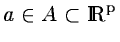are the p parameters lying in the domain A. If A is not the whole space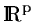, the problem is said to be constrained.

If a situation can be observed by a set of events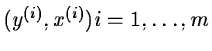, i.e. a set of couples representing the measured dependant and variables, it is possible to deduce the value of the parameters of your model y(x,a) corresponding to that situation. As the measurements are generally given with some error, it is impossible to get the exact value of the parameters but only an estimation of them. Estimating is in some sense finding the most likely value of the parameters. Much more events than parameters are in general necessary.

In a linear problem, if the errors on the observations have a gaussian distribution, the Maximum Likelihood Principle'' gives you the best estimate'' of the parameters as the solution of the so-called Least Squares Minimization'' that follows: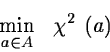withThe expected variance of the so-computed estimator is minimum among all approximation methods and is therefore called in statistics an efficient estimator''.

The quantities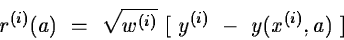are named the residuals and w(i) the weight of the ithobservation that can be, for instance, the inverse of the computed variance of the observation.

If y(x,a) depends linearly on each parameter aj, the problem is also known as a Linear Regression and is solved in MIDAS by the command REGRESSION. This chapter deals with y(x,a) which have a non-linear dependance in a.

Let us now introduce some mathematical notations. Let g(a) and H(a) be respectively the gradient and the Hessian matrix of the function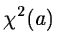. They can be expressed by

g(a) = 2 J(a)T r(a)      and

H(a) = 2 (J(a)T J(a) + B(a))

where r(a) is the residuals vector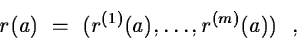J(a) the Jacobian matrix of r(a) i.e.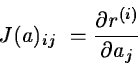and B(a) is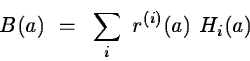with Hi(a), the Hessian matrix of r(i)(a).

In the rest of the chapter, all the functions are supposed to be differentiable if they are applied the derivation operator even when this condition is not necessary for the convergence of the algorithm.

A certain number of numerical methods have been developed to solve the non-linear least squares problem, four have so far been implemented in MIDAS. A complete description of these algorithms can be found in  and , the present document will only give a basic introduction.Next: The Newton-Raphson Method. Up: Fitting of Data Previous: Fitting of Data
http://www.eso.org/midas/midas-support.html
1999-06-09